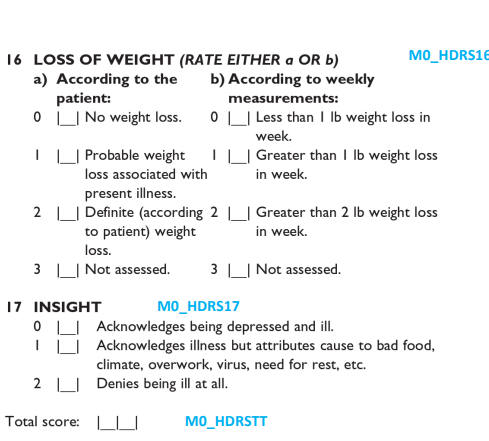# Inquiry on typing a question in excel

Hello everyone. I have a survey with 17 questions related to the Hamilton depression rating scale. As you can see in the uploaded image, question number 16 is divided into two parts a and b, and the patient should answer one of the 2 parts. It’s easy to type this question in excel but I face a problem when I want to let kobo calculate the total score (which is the summation of questions 1 to 17). So what I need now is help regarding this matter and how I should type this question in excel in a way that gives me the total score.
I hope that I was able to ask my question well.
Thank you!Welcome @nao04,
to facilitate support, would you mind to write down the logic/calculation in a textual form?
And provide more details what is not working, incl. the related part of the questionnaire?

1 Like

And this one too …

The formula of the total score is \${Q1}+ \${Q2}+\${Q3}…until +\${Q15}+\${Q16}+\${Q17}.
What I did in excel was that first I divided question 16 into 3 questions, the first one was for choosing either a or b and based on what we filled the labels of the question appears in the second and the third (I have put a relevant to let the question that we want to appear), because in the question 16 they asked us to chose either a or b.
But this way didn’t give me the total score because the formula will not be working especially if one of the questions will be hidden.
The second way that I used was to put all the labels together, but the second problem that I faced was with the name of the label. I can’t give a name 2 times for the same question (what I mean is 0 1 2 3 for a and 0 1 2 3 for b). But I should keep these names (the numbers) to be able to calculate the total score at the end.
So at the end can someone please help me in typing this question (question 16) in excel in a way that will be working and gives me the total score?

Thank you!

Actually, I don’t have a problem with the formulation of the calculation formula of the total score. My problem is with question 16 in the formula of the total score and how can I type this question in excel before I import it to kobo.

@nao04, maybe you will need to share a screenshot of Q16 so that the community should be able to help you out.

Ah, excuse me. I thought that I already uploaded it. I will do that again.

Kindly find attached the image of the questions.

Thanks

1 Like

Ah, excuse me. I thought that I already uploaded it. I will do that again.
Kindly find attached the image of the questions.
Thanks

1 Like

@nao04, have you not prepared the question in the XLSForm? That should be helpful for he community.

Frankly, I need someone help me to type this question on the XLSform in a way that gives me the total score. I didn’t be able to get it right.

@nao04, if you wish someone from the community to help you with this, maybe you could also use our job board. Our community would then be able to offer you the help to develop your form into an XLSForm.

1 Like